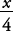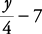## Variables and Algebraic Expressions

A variable is a symbol used to denote any element of a given set—often a letter used to stand for a number. Variables are used to change verbal expressions into algebraic expressions.

##### Example 1

Give the algebraic expression.

Verbal Expression

Algebraic Expression

The sum of a number n and 7

n + 7 or 7 + n

The number n diminished by 10

n – 10

Seven times the number n

7 n

x divided by 4Five more than the product of 2 and n

2 n + 5 or 5 + 2 n

Seven less than the quotient of y and 4